# Developing Barcode Scanner Mobile Application for iOSIvan Ozhiganov

Founder & CEO at Azoft

#Mobile

18 Feb 201618 Feb 2016

One of the projects we completed here in R&D department was to develop a barcode scanner application for iOS that could recognize even blurry barcodes, aligned in an arbitrary direction. But first, the real challenge was to come up with an algorithm to ensure we meet all requirements without sacrificing the application’s performance and quality of recognition. Here I will describe how we approached the challenge and came up with a fully-functional solution.

The project is designed as a framework, so it can be easily integrated into existing mobile applications or those currently under development.

The main goal of the project was to develop an iOS application that includes functionality for scanning linear (1D) barcodes. According to the requirements, the app should be able to scan barcodes that are not aligned along the horizontal axis and should scan them continuously. More specifically, the video stream from the mobile camera should not be interrupted, should have a rate of at least 20 frames per second, and all frames from the camera should be recognized until the device receives a signal to stop scanning.

## GPU Phase

Our approach to solving the challenge can be divided into two main stages. The first stage is the analysis and processing of input frame captured by the mobile camera in order to find and localize the barcode (i.e. determine which area of the captured frame is the barcode). The second stage is the analysis and decoding of the barcode.

The first stage requires intensive mathematical image processing. To obtain acceptable performance, we decided to use the graphics processing unit (GPU) to carry out the necessary calculations. To work with the GPU we relied on OpenGL ES 2.0 framework and used its shader model.

We used textures for data storage. Essentially, textures will serve as matrices, using which we will carry out certain operations. I must point out that one of the most labor-intensive operations for the GPU is determining the texel value of the texture. Therefore, to optimize performance we try to use all 4 channels (RGBA) of each texel for storing data.

Step 1. Convert the image captured by the camera into grayscale, since the information about color doesn’t play a role in this algorithm.

To obtain gray color we use the following terms (hereinafter programming code is in glsl language):

`float grayscale = dot(texture2D(CameraTexture, textureCoordinate).rgb, vec3(0.299, 0.587, 0.114));varying vec2 textureCoordinate;`Step 2. Image filtering can be done using a convolution method. To do this, calculate values of brightness gradient of the image in four directions: 0, 90, 45 and 135 degrees. Employ the filter in these four directions in a single pass. To save the results, use all four texture channels (R, G, B, A).

Filtering matrices:

0 degrees

-0.25    -0.25

0.25     0.25

90 degrees

0.25      -0.25

0.25      -0.25

45 degrees

0      -0.25      0

0.25     0       -0.25

0       0.25      0

135 degrees

0       0.25      0

0.25     0       -0.25

0      -0.25      0

```
varying vec2 textureCoordinate;

precision float;

uniform sampler2D CameraTexture;
uniform mat2 Matrix0;
uniform mat3 Matrix45;
uniform mat2 Matrix90;
uniform mat3 Matrix135;
uniform float Size;

void main()

{

vec4 result = vec4(0);

vec4 result00 = texture2D(CameraTexture, textureCoordinate)*3.0;
result.r += result00.r * Matrix0;
result.g += result00.r * Matrix90;

vec4 result10 = texture2D(CameraTexture, textureCoordinate-vec2(Size,0))*3.0;
result.r += result10.r * Matrix0;
result.g += result10.r * Matrix90;

result.b += result10.r * Matrix45;
result.a += result10.r * Matrix135;

vec4 result01 = texture2D(CameraTexture, textureCoordinate-vec2(0,Size))*3.0;
result.r += result01.r * Matrix0;
result.g += result01.r * Matrix90;

result.b += result01.r * Matrix45;
result.a += result01.r * Matrix135;

vec4 result11 = texture2D(CameraTexture, textureCoordinate-vec2(Size,Size))*3.0;
result.r += result11.r * Matrix0;
result.g += result11.r * Matrix90;

vec4 result12 = texture2D(CameraTexture, textureCoordinate-vec2(Size,2.0*Size))*3.0;
result.b += result12.r * Matrix45;
result.a += result12.r * Matrix135;

vec4 result21 = texture2D(CameraTexture, textureCoordinate-vec2(2.0*Size,Size))*3.0;

result.b += result21.r * Matrix45;
result.a += result21.r * Matrix135;

gl_FragColor = abs(result);
}

```

The result: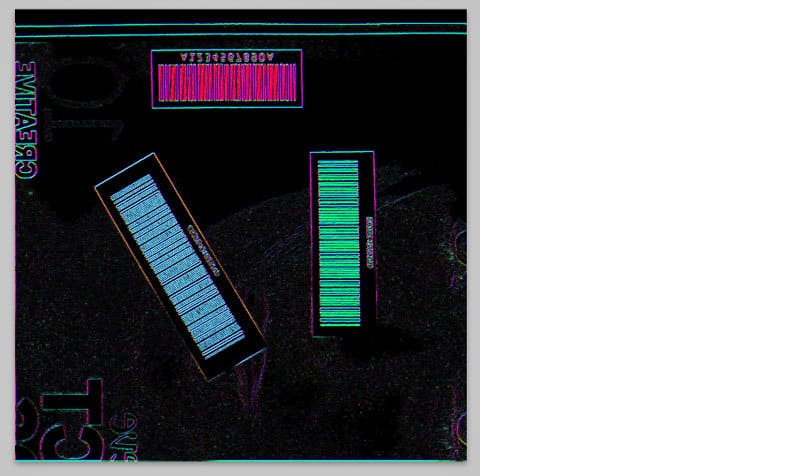Now, based on the brightness maps, we can identify which areas are probably barcodes.

Step 3. To reduce noise, filter the brightness map using Gaussian blur algorithm. To decrease the volume of calculations, let’s reduce image size two-fold. Apply Gauss filtering in 2 passes: once along the x-axis, and another time along the y-axis.

```varying highp vec2 textureCoordinate;
precision highp float;
uniform sampler2D Input;
uniform vec2 Direction; /// horizontal direction is {1.0, 0.0}; vertical direction is {0.0, 1.0}
void main()

{

vec4 sum = vec4(0);

sum += texture2D(Input, textureCoordinate - Direction*4.0) * 0.05;
sum += texture2D(Input, textureCoordinate - Direction*3.0) * 0.09;
sum += texture2D(Input, textureCoordinate - Direction*2.0) * 0.12; sum += texture2D(Input, textureCoordinate - Direction) * 0.15; sum += texture2D(Input, textureCoordinate) * 0.18;
sum += texture2D(Input, textureCoordinate + Direction) * 0.15;
sum += texture2D(Input, textureCoordinate + Direction*2.0) * 0.12;
sum += texture2D(Input, textureCoordinate + Direction*3.0) * 0.09;
sum += texture2D(Input, textureCoordinate + Direction*4.0) * 0.05;

// sum += texture2D(Input, textureCoordinate + Direction*3.0) * 0.015625;

gl_FragColor = sum;
}```

The result: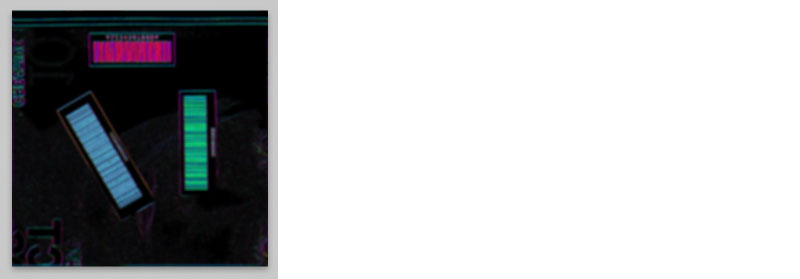Step 4. In one pass, calculate the difference between modules of gradients in orthogonal directions. A distinctive feature of barcodes is the fact that they have large enough brightness gradient in one direction, and low gradient in the orthogonal direction. Therefore, if we find the difference between orthogonal gradient across the frame, we can determine the areas of barcodes and get rid of the extra noise. Record the results into R and G channels (into the R channel we’ll record difference 0-90 and into the G channel we’ll record difference 45-135).Step 5. Convert the filtered image into a bitmap. To do this, we should compare the average brightness value with a previously set threshold. Values that are higher than the threshold should be converted to white pixels, those that are lower – to black pixels. As an intermediate result, we get an almost all-black screen with several white rectangles, which are the barcode areas.## CPU Phase

Step 1. To determine the location of each barcode in the Cartesian coordinate system, as well as to calculate their angle of inclination to the horizontal axis, we should analyze the image using algorithms of the OpenCV library. Then we find the border rectangles with minimal surface area and filter those whose surface area is lower than the specified threshold.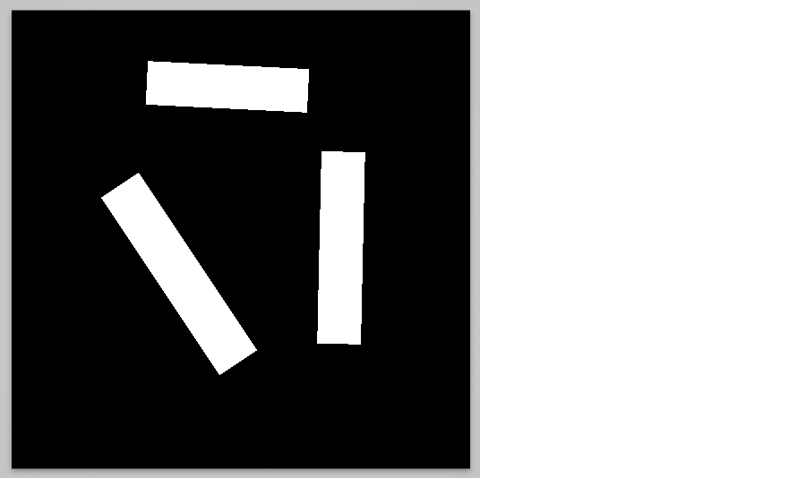Step 2. Knowing the coordinates of each barcode, we can generate new textures based on the number of barcode areas. To do this, we can cut out a portion of the original texture according to the rectangle size and align the region along the x-axis.

As a result, we get the following texture for one barcode: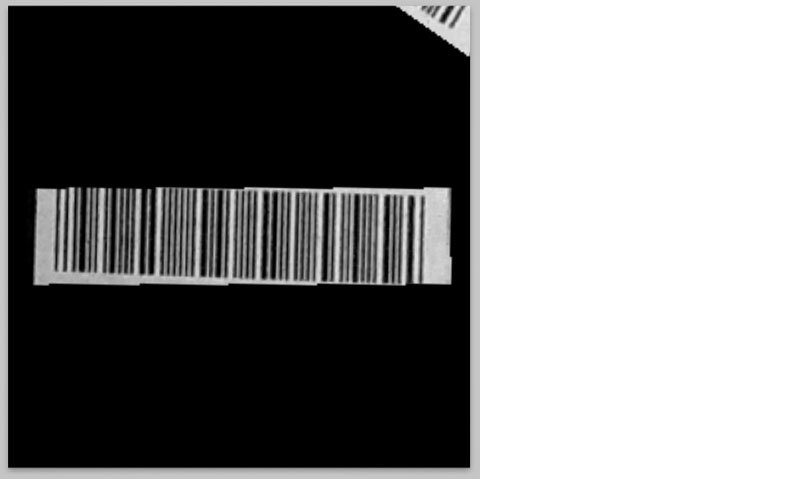So, our barcode is now properly located, centered and aligned along the x-axis. All we need to do is decipher it.

## To do this, we go back to working with the GPU:

Step 1. On the GPU we will process a single scan line from each barcode texture, examine the vector of brightness change and create a map of extremes. Then we will send the obtained data from textures of brightness maps and values of the extremes to the CPU for processing, using the object CVOpenGLESTextureCacheRef.

The result: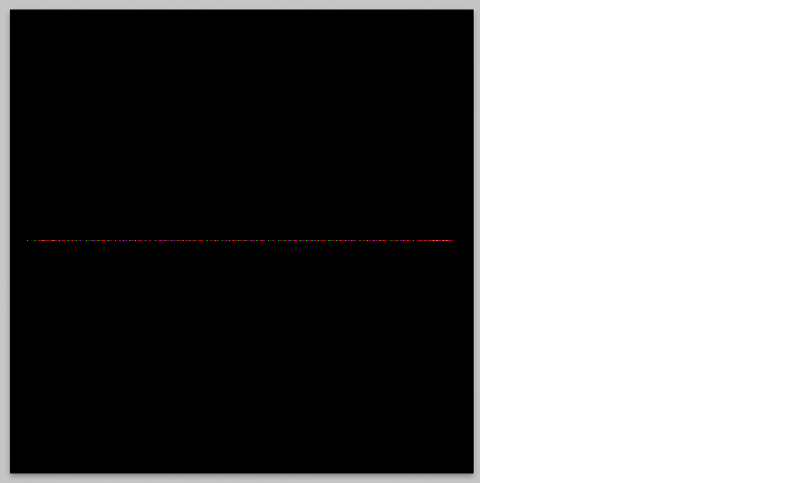Step 2. Into the R channel, we should save the brightness value of each pixel. Into the G channel, we save the highest extreme. Into the B channel, we save the lowest extreme.

Step 3. The resulting texture should be sent to the CPU, where the final analysis will take place, followed by the barcode decoding.

Using this method we can automatically align and read an unlimited number of barcodes, arranged in an arbitrary order. This way the application performance and quality of recognition aren’t compromised.

As I already mentioned before, this algorithm can detect even blurry barcodes, which is undoubtedly a great advantage, since not all mobile devices have a camera with adjustable focus.

For example, our algorithm can be used to read the following barcodes:Lastly, I’d like to say a few words about the technical characteristics of the app we developed:

• As of now, we have implemented the reading of barcodes of CODABAR, CODE39, and ISBN standards. However, because our prototype is scalable and modular, you can easily connect your algorithms to recognize other barcode formats.
• Application performance on iPhone 4 is up to 20 recognition operations per second, the figures are even higher for iPhone 4S.
• A current version of our prototype runs on iPhone 4, iPhone 4S, iPad 2, and cameras with fixed or variable focus.

In the future, we plan to expand the list of platforms that support our application. Since we used open-source and cross-platform technologies such as OpenGL 2.0 and OpenCV, it will not be difficult to move the prototype to other platforms. Moreover, in the future we hope to improve the algorithm:

• Use multiple scan lines for analysis.
• Enable EXIF-data of each frame for pre-image preparations and use other types of optimization to increase the quality and speed of reading.